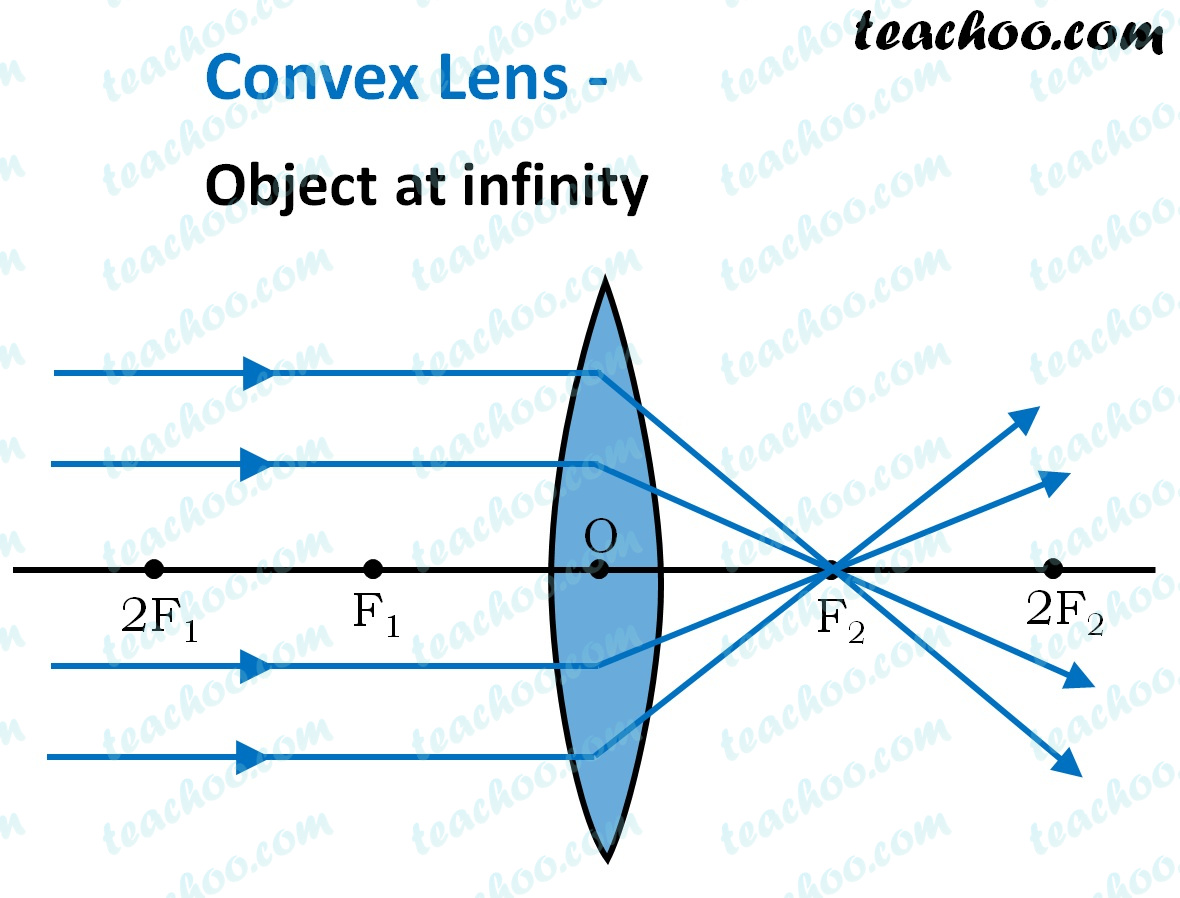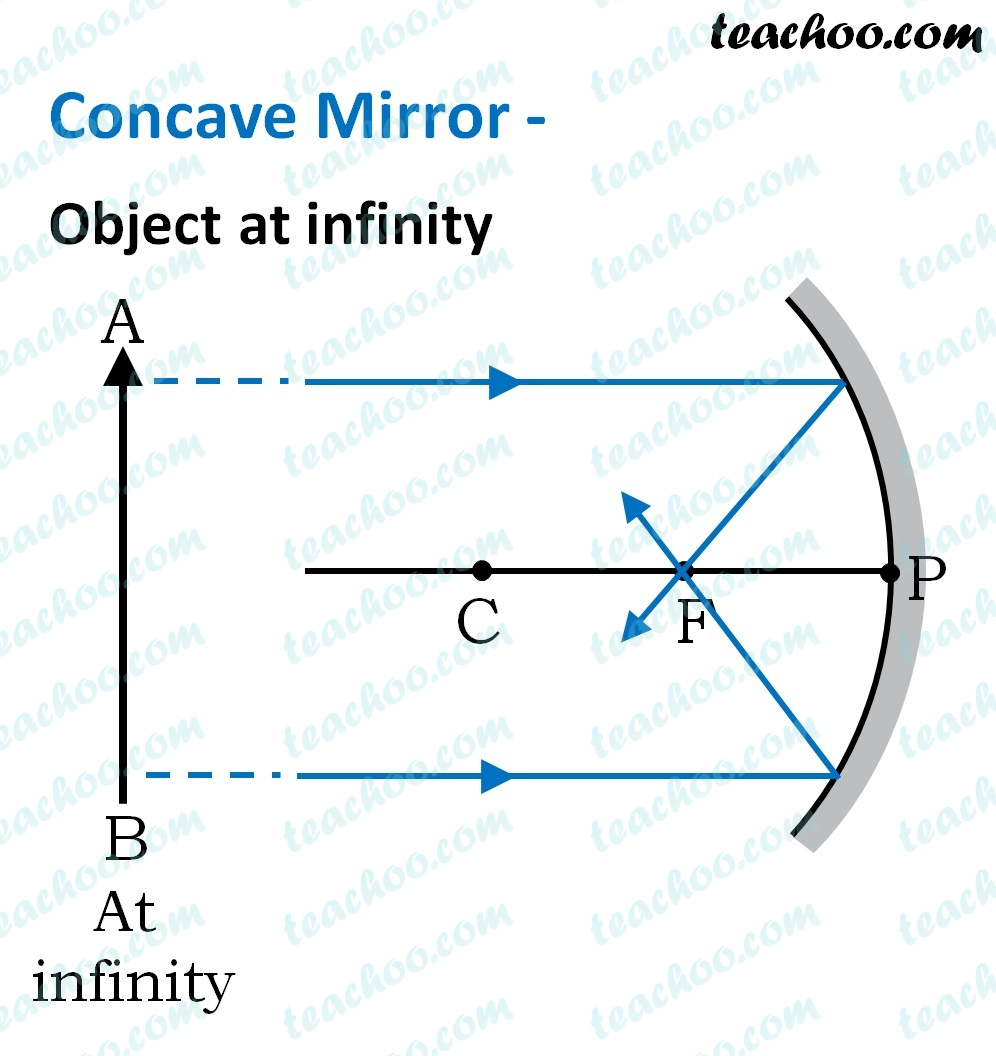## (D) Concave mirror as well as concave lens.

Only converging lens and converging mirror can make a parallel beam of light incident on a point.

• Concave mirror is a converging mirror.
• Convex lenses converge to light rays, hence convex lenses are also called converging lenses .So, the correct answer is (a) - Concave mirror as well as convex lens.

1. Class 10
2. Solutions to CBSE Sample Paper - Science Class 10
3. Solutions - CBSE Class 10 Sample Paper for 2022 Boards - Science [MCQ]

Solutions - CBSE Class 10 Sample Paper for 2022 Boards - Science [MCQ]

Class 10
Solutions to CBSE Sample Paper - Science Class 10
• Solutions - CBSE Class 10 Sample Paper for 2022 Boards - Science [MCQ]